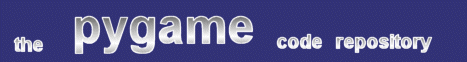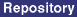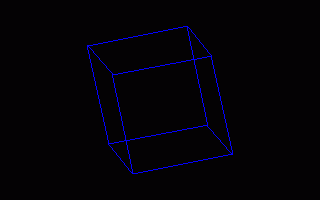### Title: 3-D Wireframe example

Author: Gustavo Niemeyer (niemeyer at conectiva.com)
Submission date: December 2, 2001

Description: 3-D Wireframe graphics example. Mr. Niemeyer uses a draw_3dline function to render a 3-d wireframe object without 3-d hardware or opengl. The result is reminiscent of the graphics of the earliest Elite games. A cube is rendered, then spun about its origin.pygame version required: 0.9 or later
SDL version required: Any
Python version required: 2.0 or later

Comments: To define a given solid, you'll have to specify your edges. This script does so by creating a list of verticies, where each vertex is a xyz coordinate. It then calls a function which defines edges between certain vertecies, and draws them. The rotate object function is more general, and takes transformation parameters similar to those used by OpenGL.

```
#!/usr/bin/python
"""
This example shows a simple wireframe rotating cube.

Written by Gustavo Niemeyer <niemeyer@conectiva.com>.
"""
from pygame.locals import *
import pygame.draw
import pygame.time
from math import sin, cos

ORIGINX = 0
ORIGINY = 0

def draw_3dline(surface, color, a, b):
"""Convert 3D coordinates to 2D and draw line."""
ax, ay = a+(a*0.3)+ORIGINX, a+(a*0.3)+ORIGINY
bx, by = b+(b*0.3)+ORIGINX, b+(b*0.3)+ORIGINY
pygame.draw.line(surface, color, (ax, ay), (bx, by))

def draw_cube(surface, color, cube):
"""Draw 3D cube."""
a, b, c, d, e, f, g, h = cube
draw_3dline(surface, color, a, b)
draw_3dline(surface, color, b, c)
draw_3dline(surface, color, c, d)
draw_3dline(surface, color, d, a)

draw_3dline(surface, color, e, f)
draw_3dline(surface, color, f, g)
draw_3dline(surface, color, g, h)
draw_3dline(surface, color, h, e)

draw_3dline(surface, color, a, e)
draw_3dline(surface, color, b, f)
draw_3dline(surface, color, c, g)
draw_3dline(surface, color, d, h)

def rotate_3dpoint(p, angle, axis):
"""Rotate a 3D point around given axis."""
ret = [0, 0, 0]
cosang = cos(angle)
sinang = sin(angle)
ret += (cosang+(1-cosang)*axis*axis)*p
ret += ((1-cosang)*axis*axis-axis*sinang)*p
ret += ((1-cosang)*axis*axis+axis*sinang)*p
ret += ((1-cosang)*axis*axis+axis*sinang)*p
ret += (cosang+(1-cosang)*axis*axis)*p
ret += ((1-cosang)*axis*axis-axis*sinang)*p
ret += ((1-cosang)*axis*axis-axis*sinang)*p
ret += ((1-cosang)*axis*axis+axis*sinang)*p
ret += (cosang+(1-cosang)*axis*axis)*p
return ret

def rotate_object(obj, angle, axis):
"""Rotate an object around given axis."""
for i in range(len(obj)):
obj[i] = rotate_3dpoint(obj[i], angle, axis)

def main():
global ORIGINX, ORIGINY
pygame.init()
screen = pygame.display.set_mode((320,200))
# Move origin to center of screen
ORIGINX = screen.get_width()/2
ORIGINY = screen.get_height()/2
cube = [(-50,50,50),  (50,50,50),  (50,-50,50),  (-50,-50,50),
(-50,50,-50), (50,50,-50), (50,-50,-50), (-50,-50,-50)]
while 1:
draw_cube(screen, 255, cube)
event = pygame.event.poll()
if event.type == QUIT or (event.type == KEYDOWN and event.key == K_ESCAPE):
break
pygame.display.flip()
pygame.time.delay(25)
draw_cube(screen, 0, cube)
rotate_object(cube, 0.1, (0,1,0))
rotate_object(cube, 0.01, (0,0,1))
rotate_object(cube, 0.01, (1,0,0))

if __name__ == "__main__":
main()

```
 From: sebastian Date: September 06, 2002 21:20 GMT with this semi-3d, is there any possibility to make the lines darker as they go away from the viewer? to get a better 3d effect? or do i have to use (py)opengl for this? thanks sebastian ps: the wireframe demo is great :)

 From: bacchus Date: February 04, 2003 03:16 GMT My first reaction, being someone who knows absolutely nothing about 3d programming, is to measure the distance of each point from the viewer in a 3d-friendly way. Set up some arbitrary ratio where, for instance, if the point is 10 units away, the color of the pixel at that point is 20 shades darker (subtract R, G, and B values by 20). Implement this with a shaded line-drawing algorithm that slowly gradients the pixels it draws to the darkest color. This wouldn't deal with the issues involving overlapping pixels, but I'm really not sure how to correctly deal with depth issues in 3d yet... maybe someone more erudite will post...

 From: Crusoe Date: September 03, 2003 15:46 GMT I wish this stuff existed in 1995! Heck, I was writing simulations of 3D bodies back then using GWBASIC, and it sucked. The lack of standard libraries, accel, etc on Linux prevented me from enjoying all this more. Now, with Pygame, sane 3d support, and SDL, I'm itching to take a crack with games!

 From: Anonymous Date: June 09, 2004 14:58 GMT Thanks

 From: nexus Date: June 17, 2004 06:16 GMT Awsome 3d wireframe model. Must have tooken along tmie for the math bx, by = b+(b*0.3)+ORIGINX, b+(b*0.3)+ORIGINY

 Feedback